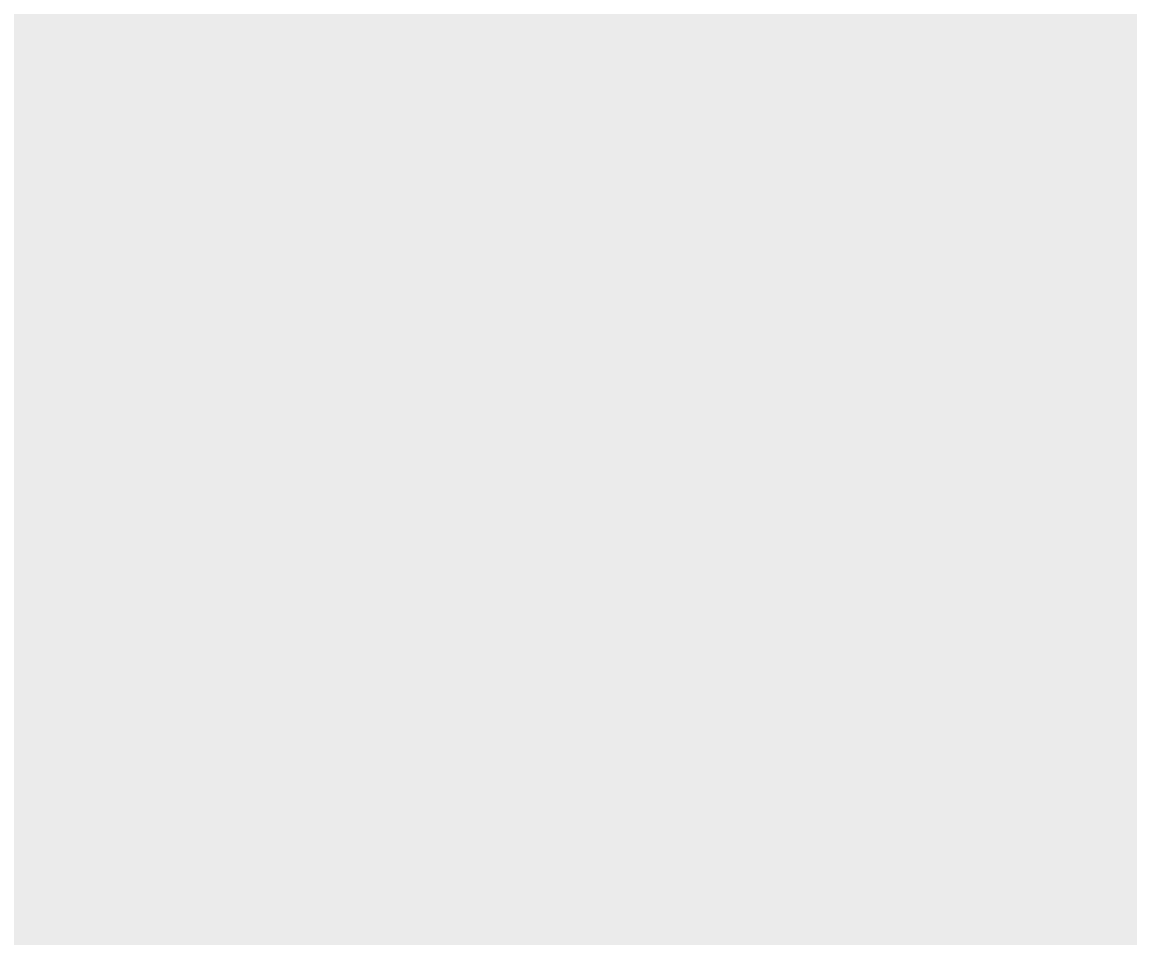## Agenda

• understand the philosophy of Grammar of Graphics
• explore different aspects of ggplot2
• learn to build some of the basic plots regularly used for exploring data

## Introduction

ggplot2 is an alternative (and awesome) to base R for data visualization. It is based on The Grammar of Graphics. In this post, we will understand the philosophy behind ggplot2 and learn to build some of the most frequently used plots for visualizing data.

## Libraries

``library(ggplot2)``
``## Warning: package 'ggplot2' was built under R version 3.5.1``

## Grammar of Graphics

Grammar of Graphics is a formal system for building plots. The core idea is that any plot can be uniquely described as a combination of

• a dataset
• a geom
• a set of mappings
• a statistic
• a coordinate system
• a faceting scheme

## Data

``ggplot(data = mtcars)``## Geom

``````ggplot(data = mtcars) +
geom_point(mapping = aes(x = disp, y = mpg))``````